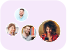Product Edition:9th Edition
Author: Robert V. Hogg
Book Name: Probability and Statistical Inference, Global Edition
Subject Name: Maths

# Probability and Statistical Inference, Global Edition 9th Edition Solutions

0 out of 5.0
57 reviews34 Students
have requested for homework help from this book

For a one- or two-semester coursecalculus background presumed no previous study of probability or statistics is required.�Written by three veteran statisticians this applied introduction to probability and statistics emphasizes the existence of variation in almost every process and how the study of probability and statistics helps us understand this variation. Designed for students with a background in calculus this book continues to reinforce basic mathematical concepts with numerous real-world examples and applications to illustrate the relevance of key concepts.Read more

5
29
4
11
3
7
2
6
1
4

0

## Students who viewed this book also checked out